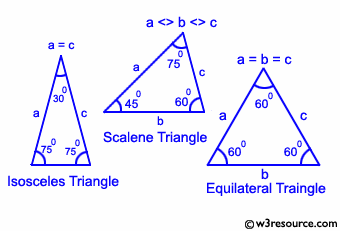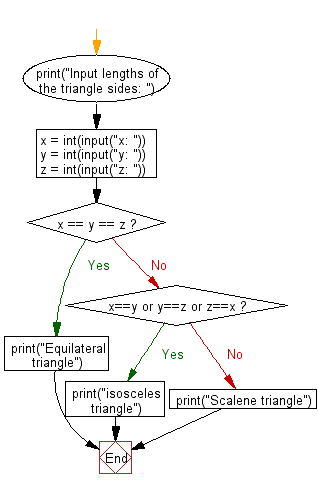﻿ Python Exercise: Check a triangle is equilateral, isosceles or scalene - w3resource# Python Exercise: Check a triangle is equilateral, isosceles or scalene

## Python Conditional: Exercise - 36 with Solution

Write a Python program to check a triangle is equilateral, isosceles or scalene.
Note :
An equilateral triangle is a triangle in which all three sides are equal.
A scalene triangle is a triangle that has three unequal sides.
An isosceles triangle is a triangle with (at least) two equal sides.

Pictorial Presentation:Sample Solution:

Python Code:

``````print("Input lengths of the triangle sides: ")
x = int(input("x: "))
y = int(input("y: "))
z = int(input("z: "))

if x == y == z:
print("Equilateral triangle")
elif x==y or y==z or z==x:
print("isosceles triangle")
else:
print("Scalene triangle")
```
```

Sample Output:

```x: 6
y: 8
z: 12
Scalene triangle
```

Flowchart :## Visualize Python code execution:

The following tool visualize what the computer is doing step-by-step as it executes the said program:

Python Code Editor:

Have another way to solve this solution? Contribute your code (and comments) through Disqus.

What is the difficulty level of this exercise?

Test your Python skills with w3resource's quiz

﻿

## Python: Tips of the Day

Returns a list with n elements removed from the beginning

Example:

```def tips_take(itr, n = 1):
return itr[:n]
print(tips_take([1, 2, 3], 5))
print(tips_take([1, 2, 3], 0))
```

Output:

```[1, 2, 3]
[]
```